# findobj

Find graphics objects with specific properties

## Syntax

``h = findobj``
``h = findobj(prop,value)``
``h = findobj('-not',prop,value)``
``h = findobj(prop1,value1,oper,prop2,value2)``
``h = findobj('-regexp',prop,expr)``
``h = findobj('-property',prop)``
``h = findobj(prop1,value1,...,propN,valueN)``
``h = findobj(objhandles,___) ``
``h = findobj(objhandles,'-depth',d,___)``
``h = findobj(objhandles,'flat',___) ``

## Description

example

````h = findobj` returns the graphics root object and all of its descendants.```

example

````h = findobj(prop,value)` returns all objects in the hierarchy that have their property `prop` set to `value`.```

example

````h = findobj('-not',prop,value)` returns all objects whose specified property is not set to the specified value.```

example

````h = findobj(prop1,value1,oper,prop2,value2)` applies the logical operator `oper` to the `prop,value` pairs. For example, `h = findobj('LineStyle','--','-and','Marker','o')` returns all objects that have a dashed line style and circular markers.```

example

````h = findobj('-regexp',prop,expr)` uses a regular expression to find objects with specific property values. Objects with property values satisfying the regular expression are returned.```

example

````h = findobj('-property',prop)` returns all objects that have the specified property.```

example

````h = findobj(prop1,value1,...,propN,valueN)` returns all objects in the hierarchy that have the specified properties set to the specified values. You can replace `prop,value` pairs with other input argument combinations from the previous syntaxes. For example, ```h = findobj(prop1,value1,'-not',prop2,value2,'-property',prop3)``` returns all objects that satisfy these three conditions: The object has a property `prop1` set to `value1`.The object has a property `prop2` whose value is not set to `value2`.The object has a property `prop3`. ```

example

````h = findobj(objhandles,___) ` restricts the search to the objects listed in `objhandles` and all of their descendants. You can restrict the search for any of the previous syntaxes.```

example

````h = findobj(objhandles,'-depth',d,___)` restricts the search to the objects listed in `objhandles` and their descendants that are up to `d` levels lower in the graphics object hierarchy.```

example

````h = findobj(objhandles,'flat',___) ` restricts the search to the objects listed only in `objhandles`. The descendant objects are not searched. Using the `'flat'` option is the same as using the `'-depth'` option with `d = 0`.```

## Examples

collapse all

Delete all existing figures, and then create a plot of random values.

```close all plot(rand(5))```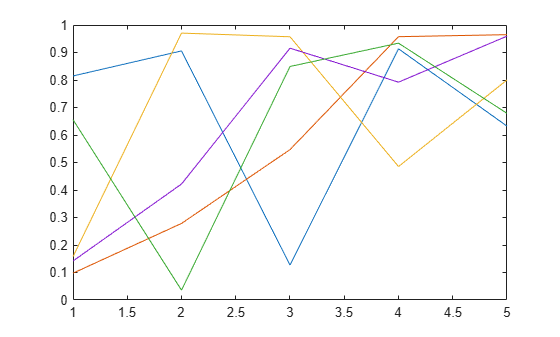Return the graphics root object and all of its descendants.

`h = findobj`
```h = 8x1 graphics array: Root Figure (1) Axes Line Line Line Line Line ```

Delete all existing figures, and then create a multiline plot.

```close all plot(magic(4))```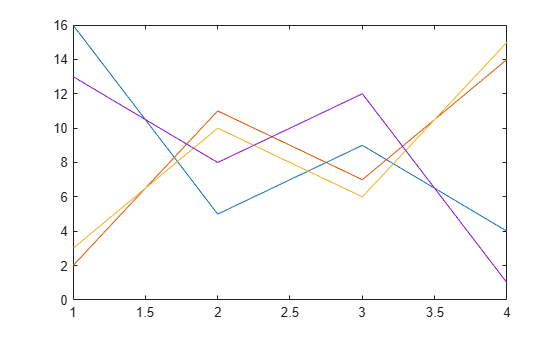Return all line objects.

`h = findobj('Type','line')`
```h = 4x1 Line array: Line Line Line Line ```

Plot nine sine waves with custom colors and line styles.

```x = linspace(0,7); y = ones(length(x),9); for i = 1:9 y(:,i) = sin(x-i/5)'; end plot(x,y) colororder({'red','green','blue'}) ax = gca; ax.LineStyleOrder = {'-','--',':'};```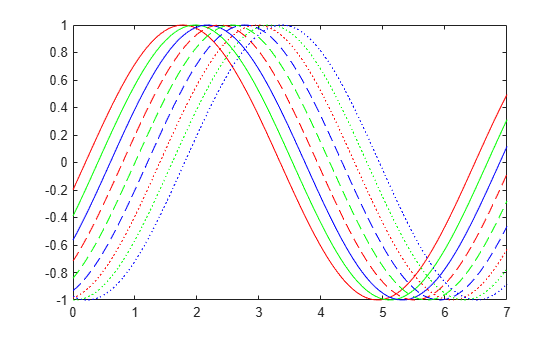Return the solid red line. Then, change the thickness of the line.

`h = findobj('Color','red','LineStyle','-')`
```h = Line with properties: Color: [1 0 0] LineStyle: '-' LineWidth: 0.5000 Marker: 'none' MarkerSize: 6 MarkerFaceColor: 'none' XData: [1x100 double] YData: [1x100 double] ZData: [1x0 double] Show all properties ```
`h.LineWidth = 2;`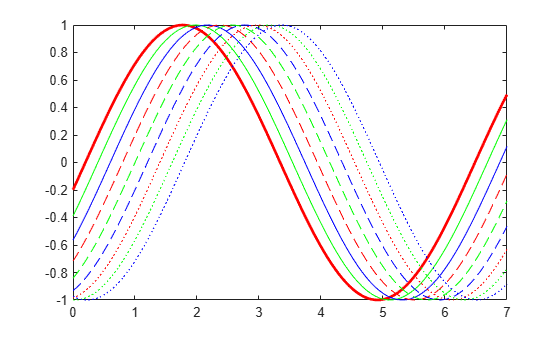Create a multiline plot. Specify an identifier for each plot.

```x = linspace(-1,1); y1 = x; plot(x,y1,'Tag','linear') hold on y2 = x.^2; plot(x,y2,'Tag','quadratic') y3 = exp(x); plot(x,y3,'Tag','exponential') y4 = sin(x); plot(x,y4,'Tag','sinusoidal') hold off```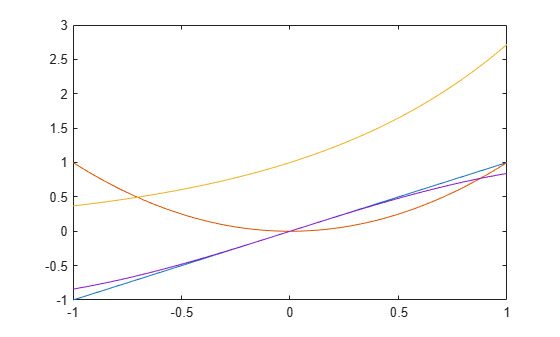Find all objects whose `Tag` property is not set to `'linear'`.

`h1 = findobj('-not','Tag','linear')`
```h1 = 6x1 graphics array: Root Figure (1) Axes Line (sinusoidal) Line (exponential) Line (quadratic) ```

Find all objects whose `Tag` property is not set to `'linear'` or `'quadratic'`.

`h2 = findobj('-not',{'Tag','linear','-or','Tag','quadratic'})`
```h2 = 5x1 graphics array: Root Figure (1) Axes Line (sinusoidal) Line (exponential) ```

Find all line objects whose `Tag` property is not set to `'linear'` or `'quadratic'`.

`h3 = findobj('Type','line','-not',{'Tag','linear','-or','Tag','quadratic'})`
```h3 = 2x1 Line array: Line (sinusoidal) Line (exponential) ```

Improve the readability of the previous statement by using `'-and'` and curly brackets.

`h4 = findobj({'Type','line'},'-and',{'-not',{'Tag','linear','-or','Tag','quadratic'}})`
```h4 = 2x1 Line array: Line (sinusoidal) Line (exponential) ```

Create three line plots and assign an identifier to two of the plots.

```x = linspace(-1,1); y1 = x; plot(x,y1) hold on y2 = x.^2; plot(x,y2,'Tag','Quadratic') y3 = exp(x); plot(x,y3,'Tag','Exponential') hold off```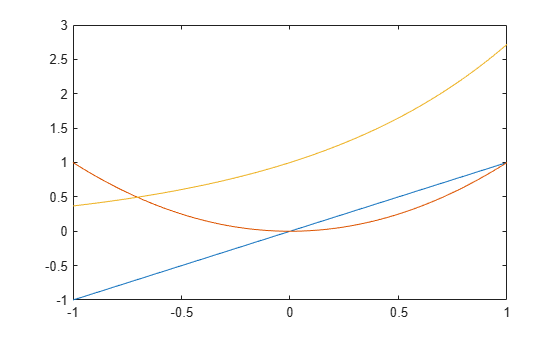Find all objects that have a nonempty `Tag` property.

`h = findobj('-regexp','Tag','[^'']')`
```h = 2x1 Line array: Line (Exponential) Line (Quadratic) ```

Create a vector of four values. Display the values using a line plot, an area plot, and a bar graph.

```y = [1 5 6 3]; subplot(3,1,1) plot(y) subplot(3,1,2) area(y) subplot(3,1,3) bar(y)```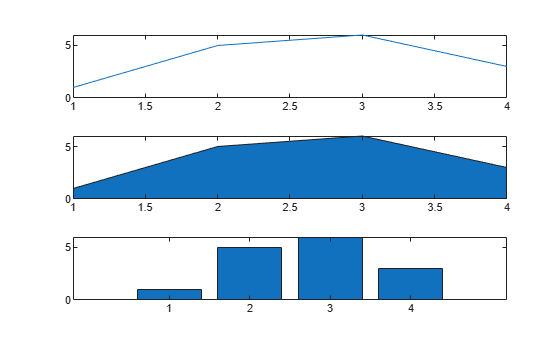Return all objects that have a `BaseValue` property.

`h = findobj('-property','BaseValue')`
```h = 2x1 graphics array: Bar Area ```

Create a plot of random values, and then return all line objects in the current axes.

`plot(rand(5))``h = findobj(gca,'Type','line')`
```h = 5x1 Line array: Line Line Line Line Line ```

Use `h` to query the y values of the first `Line` object.

`values = h(1).YData`
```values = 1×5 0.6557 0.0357 0.8491 0.9340 0.6787 ```

Create a figure with two tabs. Add axes to each tab by specifying the parent container for each one. Plot a line in the first tab and a surface in the second tab.

```figure tab1 = uitab('Title','Tab1'); ax1 = axes(tab1); plot(ax1,1:10) tab2 = uitab('Title','Tab2'); ax2 = axes(tab2); surf(ax2,peaks)```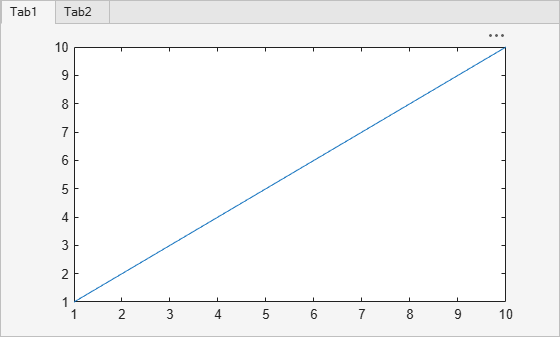Return all objects in the current figure and its descendants.

`h = findobj(gcf)`
```h = 8x1 graphics array: Figure (1) TabGroup Tab (Tab1) Tab (Tab2) Axes Axes Line Surface ```

Create a figure with two stacked subplots.

```subplot(2,1,1) x = linspace(0,10); y1 = sin(x); plot(x,y1) subplot(2,1,2) y2 = sin(5*x); plot(x,y2)```Find all objects in the current figure and its children.

`h1 = findobj(gcf,'-depth',1)`
```h1 = 3x1 graphics array: Figure (1) Axes Axes ```

Find all objects in the current figure and any descendants that are up to two levels lower in the graphics object hierarchy.

`h2 = findobj(gcf,'-depth',2)`
```h2 = 5x1 graphics array: Figure (1) Axes Axes Line Line ```

Restrict the search to the current figure and the current axes using the `'flat'` option.

`h3 = findobj([gcf,gca],'flat')`
```h3 = 2x1 graphics array: Figure (1) Axes ```

## Input Arguments

collapse all

Property name, specified as a character vector or string scalar. For more information, see Graphics Object Properties.

Example: `'Tag'`

Example: `'Type'`

Property value, specified as a scalar or array.

Logical operator, specified as `'-and'`, `'-or'`, or `'-xor'`. Logical operator precedence follows MATLAB® precedence rules. For more information, see Operator Precedence.

To control operator precedence, group `prop`,`value` pairs within cell arrays. For example, find all objects that have a `Tag` property set to `'button one'` and a `Color` property set to a value other than `'red'` or `'blue'`:

```h = findobj('Tag','button one','-and', ... '-not',{'Color','red','-or','Color','blue'})```

Regular expression, specified as a string array, character vector, or cell array of character vectors. `expr` can contain characters, metacharacters, operators, tokens, and flags that specify patterns to match in the property value. You can use `expr` only when the property value is a string or character vector. For more information about regular expressions, see `regexp`.

Objects to search from, specified as an array of graphics objects. Unless you specify the `'-depth'` or `'flat'` options, `findobj` searches the objects in the input array `objhandles` and all of their descendants in the graphics object hierarchy.

Depth of search, specified as a nonnegative integer indicating the number of levels below any given object in the input array `objhandles`.

• `d = n` — Search `n` levels of the hierarchy below each object in `objhandles`.

• `d = 0` — Search only the same level as the objects in `objhandles`. This is equivalent to specifying the `'flat'` option.

• `d = inf` — Search all levels below the objects in `objhandles`. This is equivalent to a default search without specifying the `'-depth'` or `'flat'` options.

## Tips

• `findobj` does not return graphics objects that have the `HandleVisibility` property set to `'off'`. To return all objects in the hierarchy, including hidden objects, use the `findall` function.

• `findobj` correctly matches any legal property value. For example, this code finds all objects having a `Color` property set to `red`, `r`, or ```[1 0 0]```:

`findobj('Color','r')`

• When a graphics object is a descendant of more than one object identified in `objhandles`, MATLAB searches the object each time `findobj` encounters its handle. Therefore, implicit references to a graphics object can result in multiple returns of the object.